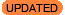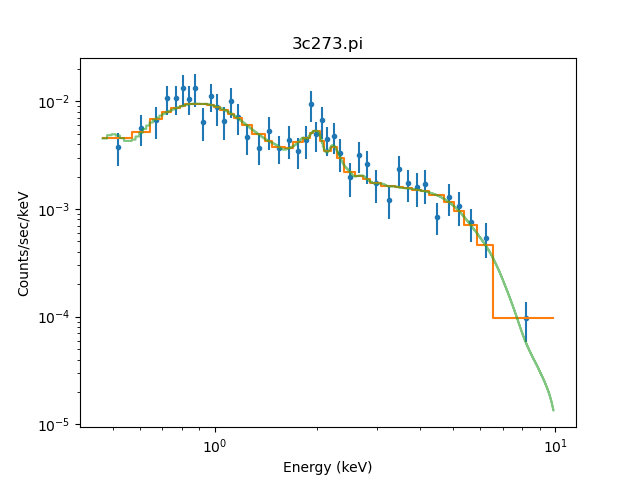# How can I plot the ungrouped fitted source model on my grouped data?New in CIAO 4.13

New in CIAO 4.13 is the ability to set the alpha transparency setting of the data with the alpha option.

When a grouped data set is fit in Sherpa, the plot_fit command plots the grouped source model overlaid on the grouped data. In order to plot the full resolution source model on the grouped data, use the plot_model command, which always plots the ungrouped source model.

For example:

```sherpa> plot_fit(xlog=True, ylog=True)
sherpa> plot_model(alpha=0.6, overplot=True)
```

creates a plot like Figure 1.

### Showing the ungrouped model### Showing the ungrouped model

The data (blue points) has been grouped to improve the signal-to-noise, and the orange line shows the model fit using this grouping scheme (created by plot_fit). The model evaluated on the instrument response by plot_model is shown as the green partially-opaque line.